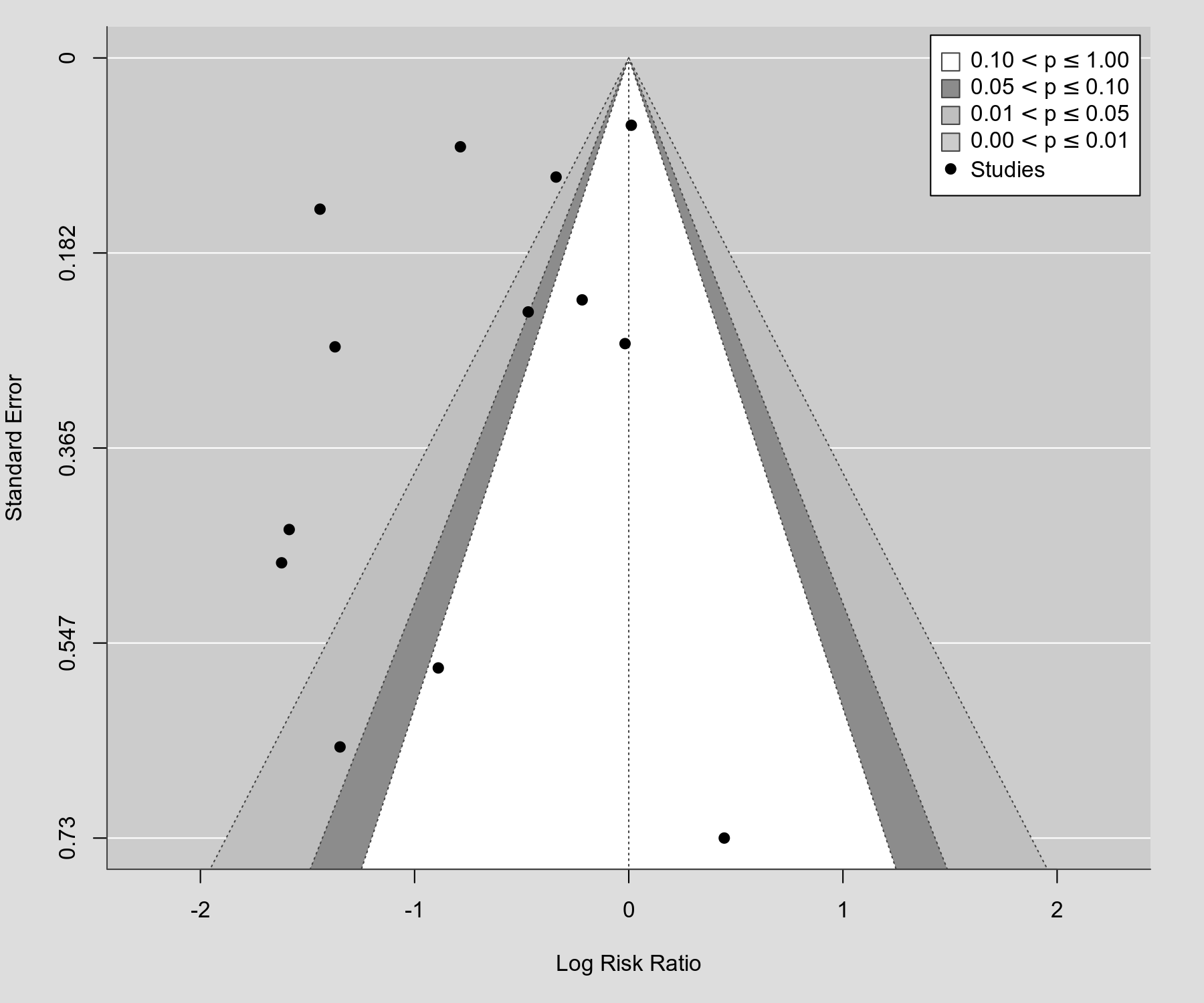#The metafor Package

A Meta-Analysis Package for R

### Sidebar

plots:contour_enhanced_funnel_plot

## Contour-Enhanced Funnel Plot

### Description

Below is an example of what is sometimes called a contour-enhanced funnel plot (Peters et al., 2008). Note that the funnel is centered not at the model estimate (as is usually done when drawing funnel plots), but at 0 (i.e., at the value under the null hypothesis of no effect). Various levels of statistical significance of the points/studies are indicated by the shaded regions. In particular, the unshaded (i.e., white) region in the middle corresponds to p-values greater than .10, the dark gray-shaded region corresponds to p-values between .10 and .05, the medium gray-shaded region corresponds to p-values between .05 and .01, and the region outside of the funnel corresponds to p-values below .01. Funnel plots drawn in this way are more useful for detecting publication bias due to the suppression of non-significant findings. See Peters et al. (2008) for more details. Note that, based on Sterne and Egger (2001), the vertical axis represents the standard error (as compared to Peters et al., 2008, who use the inverse of the standard error on the vertical axis).

### Plot### Code

library(metafor)

### decrease margins so the full space is used
par(mar=c(5,4,1,2))

### fit random-effects model
res <- rma(measure="RR", ai=tpos, bi=tneg, ci=cpos, di=cneg, data=dat.bcg)

### create contour enhanced funnel plot (with funnel centered at 0)
funnel(res, level=c(90, 95, 99), shade=c("white", "gray55", "gray75"), refline=0, legend=TRUE)

Peters, J. L., Sutton, A. J., Jones, D. R., Abrams, K. R., & Rushton, L. (2008). Contour-enhanced meta-analysis funnel plots help distinguish publication bias from other causes of asymmetry. Journal of Clinical Epidemiology, 61(10), 991–996.

Sterne, J. A. C., & Egger, M. (2001). Funnel plots for detecting bias in meta-analysis: Guidelines on choice of axis. Journal of Clinical Epidemiology, 54(10), 1046–1055.

### Page Tools